# Tag: Marcolli

At the Max-Planck Institute in Bonn Yuri Manin gave a talk about the field of one element, $\mathbb{F}_1$ earlier this week entitled “Algebraic and analytic geometry over the field F_1”.

Moreover, Javier Lopez-Pena and Bram Mesland will organize a weekly “F_un Study Seminar” starting next tuesday.

Over at Noncommutative Geometry there is an Update on the field with one element pointing us to a YouTube-clip featuring Alain Connes explaining his paper with Katia Consani and Matilde Marcolli entitled “Fun with F_un”. Here’s the clip

Finally, as I’ll be running a seminar here too on F_un, we’ve set up a group blog with the people from MPI (clearly, if you are interested to join us, just tell!). At the moment there are just a few of my old F_un posts and a library of F_un papers, but hopefully a lot will be added soon. So, have a look at F_un mathematics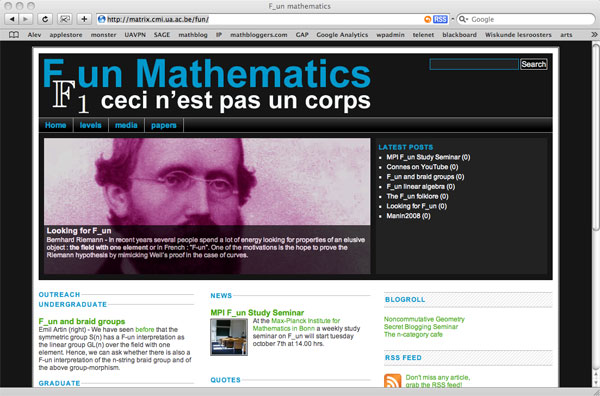There are only a handful of human activities where one goes to extraordinary lengths to keep a dream alive, in spite of overwhelming evidence : religion, theoretical physics, supporting the Belgian football team and … mathematics.

In recent years several people spend a lot of energy looking for properties of an elusive object : the field with one element $\mathbb{F}_1$, or in French : “F-un”. The topic must have reached a level of maturity as there was a conference dedicated entirely to it : NONCOMMUTATIVE GEOMETRY AND GEOMETRY OVER THE FIELD WITH ONE ELEMENT.

In this series I’d like to find out what the fuss is all about, why people would like it to exist and what it has to do with noncommutative geometry. However, before we start two remarks :

The field $\mathbb{F}_1$ does not exist, so don’t try to make sense of sentences such as “The ‘field with one element’ is the free algebraic monad generated by one constant (p.26), or the universal generalized ring with zero (p.33)” in the wikipedia-entry. The simplest proof is that in any (unitary) ring we have $0 \not= 1$ so any ring must contain at least two elements. A more highbrow version : the ring of integers $\mathbb{Z}$ is the initial object in the category of unitary rings, so it cannot be an algebra over anything else.

The second remark is that several people have already written blog-posts about $\mathbb{F}_1$. Here are a few I know of : David Corfield at the n-category cafe and at his old blog, Noah Snyder at the secret blogging seminar, Kea at the Arcadian functor, AC and K. Consani at Noncommutative geometry and John Baez wrote about it in his weekly finds.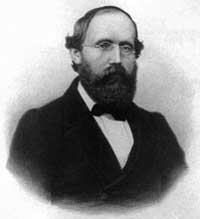The dream we like to keep alive is that we will prove the Riemann hypothesis one fine day by lifting Weil’s proof of it in the case of curves over finite fields to rings of integers.

Even if you don’t know a word about Weil’s method, if you think about it for a couple of minutes, there are two immediate formidable problems with this strategy.

For most people this would be evidence enough to discard the approach, but, we mathematicians have found extremely clever ways for going into denial.

The first problem is that if we want to think of $\mathbf{spec}(\mathbb{Z})$ (or rather its completion adding the infinite place) as a curve over some field, then $\mathbb{Z}$ must be an algebra over this field. However, no such field can exist…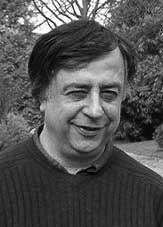No problem! If there is no such field, let us invent one, and call it $\mathbb{F}_1$. But, it is a bit hard to do geometry over an illusory field. Christophe Soule succeeded in defining varieties over $\mathbb{F}_1$ in a talk at the 1999 Arbeitstagung and in a more recent write-up of it : Les varietes sur le corps a un element.

We will come back to this in more detail later, but for now, here’s the main idea. Consider an existent field $k$ and an algebra $k \rightarrow R$ over it. Now study the properties of the functor (extension of scalars) from $k$-schemes to $R$-schemes. Even if there is no morphism $\mathbb{F}_1 \rightarrow \mathbb{Z}$, let us assume it exists and define $\mathbb{F}_1$-varieties by requiring that these guys should satisfy the properties found before for extension of scalars on schemes defined over a field by going to schemes over an algebra (in this case, $\mathbb{Z}$-schemes). Roughly speaking this defines $\mathbb{F}_1$-schemes as subsets of points of suitable $\mathbb{Z}$-schemes.

But, this is just one half of the story. He adds to such an $\mathbb{F}_1$-variety extra topological data ‘at infinity’, an idea he attributes to J.-B. Bost. This added feature is a $\mathbb{C}$-algebra $\mathcal{A}_X$, which does not necessarily have to be commutative. He only writes : “Par ignorance, nous resterons tres evasifs sur les proprietes requises sur cette $\mathbb{C}$-algebre.”The algebra $\mathcal{A}_X$ originates from trying to bypass the second major obstacle with the Weil-Riemann-strategy. On a smooth projective curve all points look similar as is clear for example by noting that the completions of all local rings are isomorphic to the formal power series $k[[x]]$ over the basefield, in particular there is no distinction between ‘finite’ points and those lying at ‘infinity’.

The completions of the local rings of points in $\mathbf{spec}(\mathbb{Z})$ on the other hand are completely different, for example, they have residue fields of different characteristics… Still, local class field theory asserts that their quotient fields have several common features. For example, their Brauer groups are all isomorphic to $\mathbb{Q}/\mathbb{Z}$. However, as $Br(\mathbb{R}) = \mathbb{Z}/2\mathbb{Z}$ and $Br(\mathbb{C}) = 0$, even then there would be a clear distinction between the finite primes and the place at infinity…

Alain Connes came up with an extremely elegant solution to bypass this problem in Noncommutative geometry and the Riemann zeta function. He proposes to replace finite dimensional central simple algebras in the definition of the Brauer group by AF (for Approximately Finite dimensional)-central simple algebras over $\mathbb{C}$. This is the origin and the importance of the Bost-Connes algebra.

We will come back to most of this in more detail later, but for the impatient, Connes has written a paper together with Caterina Consani and Matilde Marcolli Fun with $\mathbb{F}_1$ relating the Bost-Connes algebra to the field with one element.

By now, everyone remotely interested in Connes’ approach to the Riemann hypothesis, knows the _one line mantra_

one can use noncommutative geometry to extend Weil’s proof of the Riemann-hypothesis in the function field case to that of number fields

But, can one go beyond this sound-bite in a series of blog posts? A few days ago, I was rather optimistic, but now, after reading-up on the Connes-Consani-Marcolli project, I feel overwhelmed by the sheer volume of their work (and by my own ignorance of key tools in the approach). The most recent account takes up half of the 700+ pages of the book Noncommutative Geometry, Quantum Fields and Motives by Alain Connes and Matilde Marcolli…

So let us set a more modest goal and try to understand one of the first papers Alain Connes wrote about the RH : Noncommutative geometry and the Riemann zeta function. It is only 24 pages long and relatively readable. But even then, the reader needs to know about class field theory, the classification of AF-algebras, Hecke algebras, etc. etc. Most of these theories take a book to explain. For example, the first result he mentions is the main result of local class field theory which appears only towards the end of the 200+ pages of Jean-Pierre Serre’s Local Fields, itself a somewhat harder read than the average blogpost…

Anyway, we will see how far we can get. Here’s the plan : I’ll take the heart-bit of their approach : the Bost-Connes system, and will try to understand it from an algebraist’s viewpoint. Today we will introduce the groups involved and describe their cosets.

For any commutative ring $R$ let us consider the group of triangular $2 \times 2$ matrices of the form

$P_R = { \begin{bmatrix} 1 & b \\ 0 & a \end{bmatrix}~|~b \in R, a \in R^* }$

(that is, $a$ in an invertible element in the ring $R$). This is really an affine group scheme defined over the integers, that is, the coordinate ring

$\mathbb{Z}[P] = \mathbb{Z}[x,x^{-1},y]$ becomes a Hopf algebra with comultiplication encoding the group-multiplication. Because

$\begin{bmatrix} 1 & b_1 \\ 0 & a_1 \end{bmatrix} \begin{bmatrix} 1 & b_2 \\ 0 & a_2 \end{bmatrix} = \begin{bmatrix} 1 & 1 \times b_2 + b_1 \times a_2 \\ 0 & a_1 \times a_2 \end{bmatrix}$

we have $\Delta(x) = x \otimes x$ and $\Delta(y) = 1 \otimes y + y \otimes x$, or $x$ is a group-like element whereas $y$ is a skew-primitive. If $R \subset \mathbb{R}$ is a subring of the real numbers, we denote by $P_R^+$ the subgroup of $P_R$ consisting of all matrices with $a > 0$. For example,

$\Gamma_0 = P_{\mathbb{Z}}^+ = { \begin{bmatrix} 1 & n \\ 0 & 1 \end{bmatrix}~|~n \in \mathbb{Z} }$

which is a subgroup of $\Gamma = P_{\mathbb{Q}}^+$ and our first job is to describe the cosets.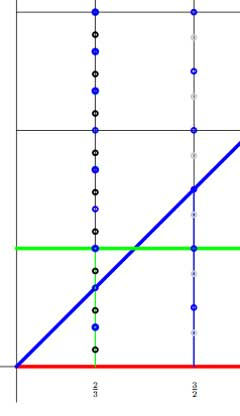The left cosets $\Gamma / \Gamma_0$ are the subsets $\gamma \Gamma_0$ with $\gamma \in \Gamma$. But,

$\begin{bmatrix} 1 & b \\ 0 & a \end{bmatrix} \begin{bmatrix} 1 & n \\ 0 & 1 \end{bmatrix} = \begin{bmatrix} 1 & b+n \\ 0 & a \end{bmatrix}$

so if we represent the matrix $\gamma = \begin{bmatrix} 1 & b \\ 0 & a \end{bmatrix}$ by the point $~(a,b)$ in the right halfplane, then for a given positive rational number $a$ the different cosets are represented by all $b \in [0,1) \cap \mathbb{Q} = \mathbb{Q}/\mathbb{Z}$. Hence, the left cosets are all the rational points in the region between the red and green horizontal lines. For fixed $a$ the cosets correspond to the rational points in the green interval (such as over $\frac{2}{3}$ in the picture on the left.

Similarly, the right cosets $\Gamma_0 \backslash \Gamma$ are the subsets $\Gamma_0 \gamma$ and as

$\begin{bmatrix} 1 & n \\ 0 & 1 \end{bmatrix} \begin{bmatrix} 1 & b \\ 0 & a \end{bmatrix} = \begin{bmatrix} 1 & b+na \\ 0 & a \end{bmatrix}$

we see similarly that the different cosets are precisely the rational points in the region between the lower red horizontal and the blue diagonal line. So, for fixed $a$ they correspond to rational points in the blue interval (such as over $\frac{3}{2}$) $[0,a) \cap \mathbb{Q}$. But now, let us look at the double coset space $\Gamma_0 \backslash \Gamma / \Gamma_0$. That is, we want to study the orbits of the action of $\Gamma_0$, acting on the right, on the left-cosets $\Gamma / \Gamma_0$, or equivalently, of the action of $\Gamma_0$ acting on the left on the right-cosets $\Gamma_0 \backslash \Gamma$. The crucial observation to make is that these actions have finite orbits, or equivalently, that $\Gamma_0$ is an almost normal subgroup of $\Gamma$ meaning that $\Gamma_0 \cap \gamma \Gamma_0 \gamma^{-1}$ has finite index in $\Gamma_0$ for all $\gamma \in \Gamma$. This follows from

$\begin{bmatrix} 1 & n \\ 0 & 1 \end{bmatrix} \begin{bmatrix} 1 & b \\ 0 & a \end{bmatrix} \begin{bmatrix} 1 & m \\ 0 & 1 \end{bmatrix} = \begin{bmatrix} 1 & b+m+an \\ 0 & a \end{bmatrix}$

and if $n$ varies then $an$ takes only finitely many values modulo $\mathbb{Z}$ and their number depends only on the denominator of $a$. In the picture above, the blue dots lying on the line over $\frac{2}{3}$ represent the double coset

$\Gamma_0 \begin{bmatrix} 1 & \frac{2}{3} \\ 0 & \frac{2}{3} \end{bmatrix}$ and we see that these dots split the left-cosets with fixed value $a=\frac{2}{3}$ (that is, the green line-segment) into three chunks (3 being the denominator of a) and split the right-cosets (the line-segment under the blue diagonal) into two subsegments (2 being the numerator of a). Similarly, the blue dots on the line over $\frac{3}{2}$ divide the left-cosets in two parts and the right cosets into three parts.

This shows that the $\Gamma_0$-orbits of the right action on the left cosets $\Gamma/\Gamma_0$ for each matrix $\gamma \in \Gamma$ with $a=\frac{2}{3}$ consist of exactly three points, and we denote this by writing $L(\gamma) = 3$. Similarly, all $\Gamma_0$-orbits of the left action on the right cosets $\Gamma_0 \backslash \Gamma$ with this value of a consist of two points, and we write this as $R(\gamma) = 2$.

For example, on the above picture, the black dots on the line over $\frac{2}{3}$ give the matrices in the double coset of the matrix

$\gamma = \begin{bmatrix} 1 & \frac{1}{7} \\ 0 & \frac{2}{3} \end{bmatrix}$

and the gray dots on the line over $\frac{3}{2}$ determine the elements of the double coset of

$\gamma^{-1} = \begin{bmatrix} 1 & -\frac{3}{14} \\ 0 & \frac{3}{2} \end{bmatrix}$

and one notices (in general) that $L(\gamma) = R(\gamma^{-1})$. But then, the double cosets with $a=\frac{2}{3}$ are represented by the rational b’s in the interval $[0,\frac{1}{3})$ and those with $a=\frac{3}{2}$ by the rational b’s in the interval $\frac{1}{2}$. In general, the double cosets of matrices with fixed $a = \frac{r}{s}$ with $~(r,s)=1$ are the rational points in the line-segment over $a$ with $b \in [0,\frac{1}{s})$.

That is, the Bost-Connes double coset space $\Gamma_0 \backslash \Gamma / \Gamma_0$ are the rational points in a horrible fractal comb. Below we have drawn only the part of the dyadic values, that is when $a = \frac{r}{2^t}$ in the unit inverval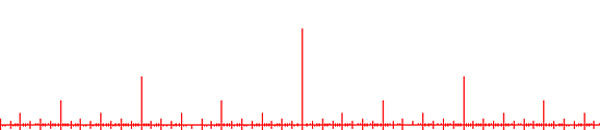and of course we have to super-impose on it similar pictures for rationals with other powers as their denominators. Fortunately, NCG excels in describing such fractal beasts…

UPDATE : here is a slightly beter picture of the coset space, drawing the part over all rational numbers contained in the 15-th Farey sequence. The blue segments of length one are at 1,2,3,…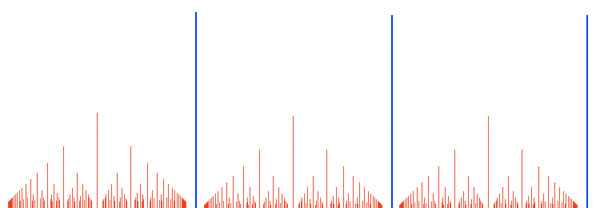MacBookAir? Is this really the best Apple could come up with? A laptop you can slide under the door or put in an envelop? Yeez… Probably the hot-air-book is about as thick as an iTouch. The first thing I did was to buy a leather case to protect the vulnerable thing, making it as thick as a first generation iPod… (needless to say, when my MacBookPro breaks down, ill replace it with a MacBookAir, clearly!)

Ranting about MacWorlds : Wired has a great article on last year’s event. Steve Job’s iPhone presentation is something that will be part of the collective memory when it comes to 2007-recollections. Few people will have realized that the Apple-team didnt have a working prototype a few weeks before… Here’s The Untold Story: How the iPhone Blew Up the Wireless Industry. A good read!

If you plug in your jailbroken iTouch, you will be asked wether you want to upgrade to 1.1.3, something we all feared for a long time and so it takes just nanoseconds to hit the cancel-button. But, there is good news! Rupert Gee reports that you can downgrade to 1.1.1 and redo jailbreak. I won’t try it for some time, but still…

In the unlikely event that you come here being a mathematician, here’s what I did with my iTouch today. Ive downloaded the Connes-Marcolli talks on Renormalization and Motives part 1, part 2, part 3, part 4, part 5, part 6, part 7 and part 8 at work. They are in mp4-format so you can load them into iTunes and onto your iTouch!!! Weather is not favorable for outdoor-cycling at the moment, so I used the home-trainer, put the iTouch in front of me and, boy, was I educated…

Here a list of saved pdf-files of previous NeverEndingBooks-posts on geometry in reverse chronological order.